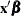# The MDC Procedure

### OUTPUT Statement

• OUTPUT options;

The OUTPUT statement creates a new SAS data set that contains all the variables in the input data set and, optionally, the estimated linear predictors (XBETA) and predicted probabilities (P). The input data set must be sorted by the choice variables within each ID.

OUT=SAS-data-set

specifies the name of the output data set.

PRED=variable name
P=variable name

requests the predicted probabilities by naming the variable that contains the predicted probabilities in the output data set.

XBETA=variable name

names the variable that contains the linear predictor () values. However, the XBETA= option is not supported in the nested logit model.